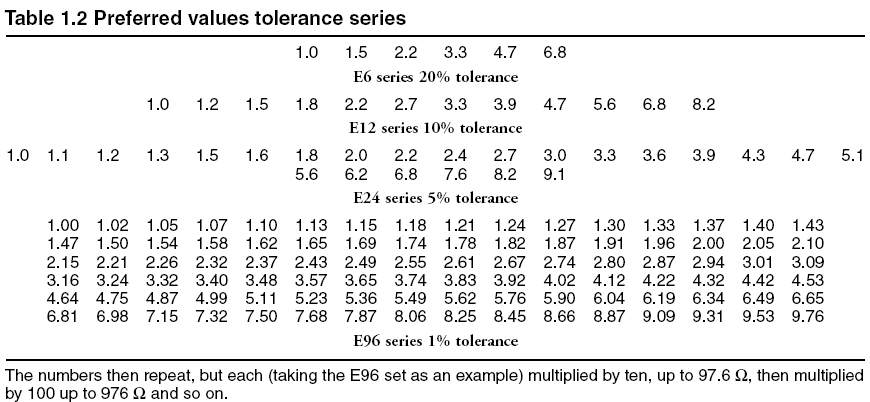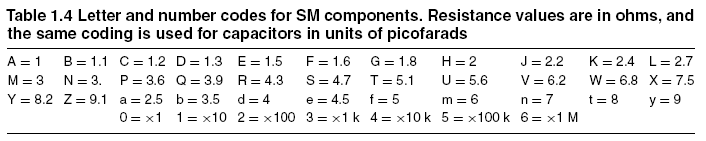Home | Gadget | Fuzzy | Lecturer  | Power  | Transceiver Find more type here... Resistor   Values of resistors (and capacitors) that use conventional wire mounting are usually indicated by a set of coloured bands (Figure 1.6). At one time, three bands were used, allowing two significant digits and one multiplier figure, but because of the widespread use of close-tolerance components, it is now more common to use four or five bands with one band used for tolerance. The use of the tolerance band is a useful guide to the order of bands, because there is often no other indication of which end of the resistor band 1 is located. In the absence of other clues, you have to assume that the correct order of bands is the one that gives value in a valid tolerance set. The coding (Table 1.3) can use three bands for value and one for tolerance for components in the tolerances from E6 to E24 (one place of decimals). In this scheme, the first band shows the first significant figure, the second band the second significant figure, and the third band the multiplier (the power of ten), with the fourth band indicating tolerance. For the E96 values, an additional significant figure band is added, so that the tolerance band is the fifth. Resistors manufactured for some specialized purposes can use an additional band to indicate temperature coefficient. Resistance values on components and in component lists are often coded according to BS 1852. In this scheme, no decimal points are used and a value in ohms is indicated by R, kilohms by K (not k), and mega ohms  by M. The letter R, K or M is used in place of the decimal point, with a zero in the leading position if the value is less than 1 ohm. This scheme avoids two sources of confusion: 1. the appearance of a dot due to a dirty photocopy being taken as adecimal point. 2. the continental practice of using commas and points in the opposite sense to UK practice. Example: 1K5 = 1.5 k or 1500 ohms; 2M2 = 2.2 M; 0R5 = 0.5 ohms.Surface mounted resistors Two forms of coding are used for surface mounted resistors (and capacitors). The three-symbol code uses two digits for the significant figures and one as multiplier, so that 471 = 47×10 = 470W and 563 = 5K6. Values below 10 are indicated in BS1852 form, so that 2R2 = 2.2W. The alternative marking, which is better suited to E96 resistors makes use of letter codes for the significant figures, and a number to indicate the multiplier. The codes are indicated in Table 1.4.   Resistor characteristics Important characteristics of resistor types include resistance ranges, usable temperature range, stability, noise level, and temperature coefficient. Wire wound resistors are available in values that range from fractions of an ohm(usually 0R22) up to about 10 kW (though higher values up to 100 kW